### Home > CALC > Chapter 10 > Lesson 10.3.1 > Problem10-113

10-113.
1. Determine if the following series converge. State the test you used. Homework Help ✎

1.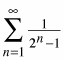2.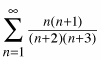3.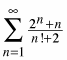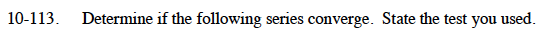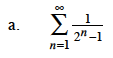How does this series compare to the following series?

$\sum_{n=1}^{\infty}\frac{1}{2^n}= \sum_{n=1}^{\infty}\Big(\frac{1}{2}\Big)^n$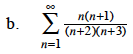This series diverges. Why?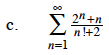$\lim_{n\to\infty}\frac{2^{n+1}+n+1}{(n+1)!+2}\cdot\frac{n!+2}{2^n+n}$